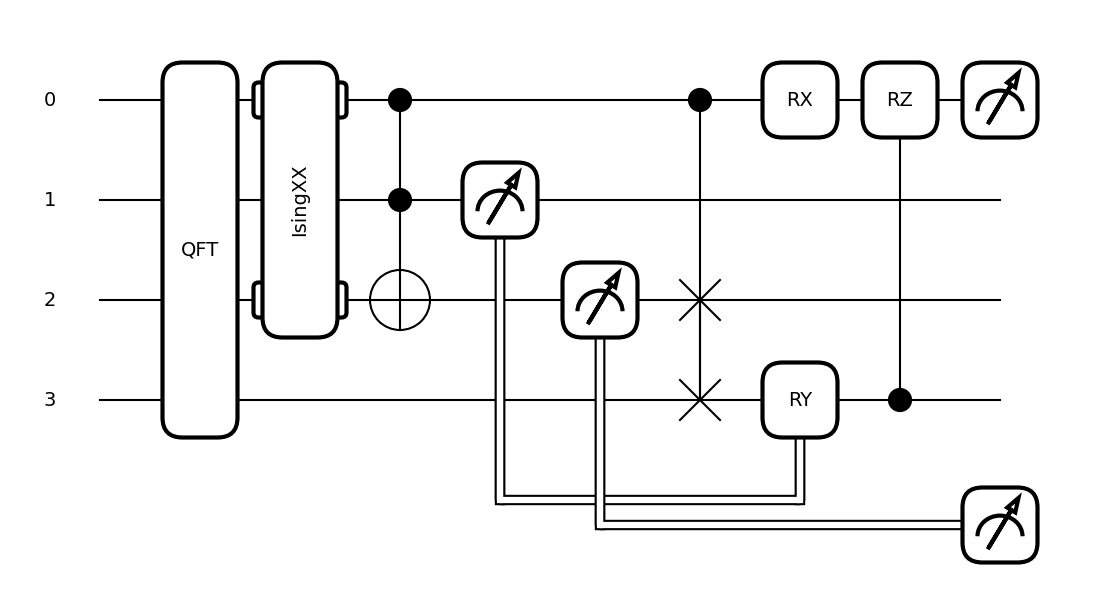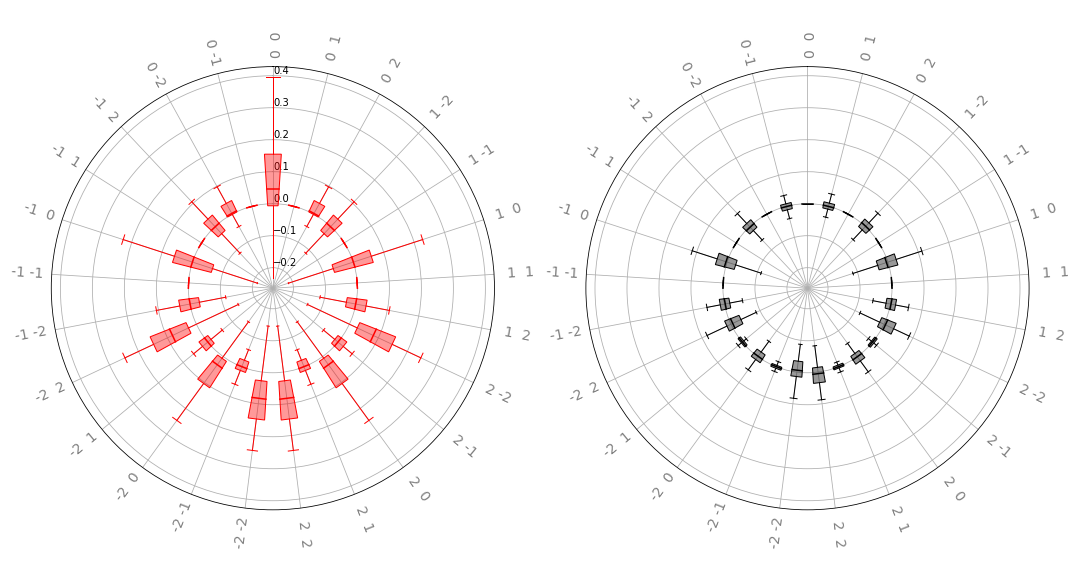# Inspecting circuits¶

PennyLane offers functionality to inspect, visualize or analyze quantum circuits.

Most of these tools are implemented as transforms. Transforms take a QNode instance and return a function:

>>> @qml.qnode(dev, diff_method='parameter-shift')
... def my_qnode(x, a=True):
...     # ...
>>> new_func = my_transform(qnode)


This new function accepts the same arguments as the QNode and returns the desired outcome, such as a dictionary of the QNode’s properties, a matplotlib figure drawing the circuit, or a DAG representing its connectivity structure.

>>> new_func(0.1, a=False)


More information on the concept of transforms can be found in Di Matteo et al. (2022).

## Extracting properties of a circuit¶

The specs() transform takes a QNode and creates a function that returns details about the QNode, including depth, number of gates, and number of gradient executions required.

For example:

dev = qml.device('default.qubit', wires=4)

@qml.qnode(dev, diff_method='parameter-shift')
def circuit(x, y):
qml.RX(x, wires=0)
qml.Toffoli(wires=(0, 1, 2))
qml.CRY(x, wires=(0, 1))
qml.Rot(x, x, y, wires=0)
return qml.expval(qml.PauliZ(0)), qml.expval(qml.PauliX(1))


We can now use the specs() transform to generate a function that returns details and resource information:

>>> x = np.array([0.05, 0.1, 0.2, 0.3], requires_grad=True)
>>> specs_func = qml.specs(circuit)
>>> specs_func(x, y)
{'resources': Resources(num_wires=3, num_gates=4, gate_types=defaultdict(<class 'int'>, {'RX': 1, 'Toffoli': 1, 'CRY': 1, 'Rot': 1}), depth=4, shots=0),
'gate_sizes': defaultdict(int, {1: 2, 3: 1, 2: 1}),
'gate_types': defaultdict(int, {'RX': 1, 'Toffoli': 1, 'CRY': 1, 'Rot': 1}),
'num_operations': 4,
'num_observables': 2,
'num_diagonalizing_gates': 1,
'num_used_wires': 3,
'num_trainable_params': 4,
'depth': 4,
'num_device_wires': 4,
'device_name': 'default.qubit',
'interface': 'auto',
'diff_method': 'parameter-shift',


## Circuit drawing¶

PennyLane has two built-in circuit drawers, draw() and draw_mpl().

For example:

dev = qml.device('lightning.qubit', wires=(0,1,2,3))

@qml.qnode(dev)
def circuit(x, z):
qml.QFT(wires=(0,1,2,3))
qml.IsingXX(1.234, wires=(0,2))
qml.Toffoli(wires=(0,1,2))
qml.CSWAP(wires=(0,2,3))
qml.RX(x, wires=0)
qml.CRZ(z, wires=(3,0))
return qml.expval(qml.PauliZ(0))

fig, ax = qml.draw_mpl(circuit)(1.2345,1.2345)
fig.show()>>> print(qml.draw(circuit)(1.2345,1.2345))
0: ─╭QFT─╭IsingXX(1.23)─╭●─╭●─────RX(1.23)─╭RZ(1.23)─┤  <Z>
1: ─├QFT─│──────────────├●─│───────────────│─────────┤
2: ─├QFT─╰IsingXX(1.23)─╰X─├SWAP───────────│─────────┤
3: ─╰QFT───────────────────╰SWAP───────────╰●────────┤


More information, including various fine-tuning options, can be found in the drawing module.

## Debugging with mid-circuit snapshots¶

When debugging quantum circuits run on simulators, we may want to inspect the current quantum state between gates.

Snapshot is an operator like a gate, but it saves the device state at its location in the circuit instead of manipulating the quantum state.

Currently supported devices include:

• default.qubit: each snapshot saves the quantum state vector

• default.mixed: each snapshot saves the density matrix

• default.gaussian: each snapshot saves the covariance matrix and vector of means

During normal execution, the snapshots are ignored:

dev = qml.device("default.qubit", wires=2)

@qml.qnode(dev, interface=None)
def circuit():
qml.Snapshot()
qml.Snapshot("very_important_state")
qml.CNOT(wires=[0, 1])
qml.Snapshot()
return qml.expval(qml.PauliX(0))


However, when using the snapshots() transform, intermediate device states will be stored and returned alongside the results.

>>> qml.snapshots(circuit)()
{0: array([1.+0.j, 0.+0.j, 0.+0.j, 0.+0.j]),
'very_important_state': array([0.707+0.j, 0.+0.j, 0.707+0.j, 0.+0.j]),
2: array([0.707+0.j, 0.+0.j, 0.+0.j, 0.707+0.j]),
'execution_results': array(0.)}


## Graph representation¶

PennyLane makes use of several ways to represent a quantum circuit as a Directed Acyclic Graph (DAG).

### DAG of causal relations between ops¶

A DAG can be used to represent which operator in a circuit is causally related to another. There are two options to construct such a DAG:

The CircuitGraph class takes a list of gates or channels and hermitian observables as well as a set of wire labels and constructs a DAG in which the Operator instances are the nodes, and each directed edge corresponds to a wire (or a group of wires) on which the “nodes” act subsequently.

For example, this can be used to compute the effective depth of a circuit, or to check whether two gates causally influence each other.

import pennylane as qml
from pennylane import CircuitGraph

dev = qml.device('lightning.qubit', wires=(0,1,2,3))

@qml.qnode(dev)
def circuit():
qml.CNOT([1, 2])
qml.CNOT([2, 3])
qml.CNOT([3, 1])
return qml.expval(qml.PauliZ(0))

circuit()
tape = circuit.qtape
ops = tape.operations
obs = tape.observables
g = CircuitGraph(ops, obs, tape.wires)


Internally, the CircuitGraph class constructs a rustworkx graph object.

>>> type(g.graph)
<class 'rustworkx.PyDiGraph'>


There is no edge between the Hadamard and the first CNOT, but between consecutive CNOT gates:

>>> g.has_path(ops, ops)
False
>>> g.has_path(ops, ops)
True


The Hadamard is connected to the observable, while the CNOT operators are not. The observable does not follow the Hadamard.

>>> g.has_path(ops, obs)
True
>>> g.has_path(ops, obs)
False
>>> g.has_path(obs, ops)
False


Another way to construct the “causal” DAG of a circuit is to use the tape_to_graph() function used by the qcut module. This function takes a quantum tape and creates a MultiDiGraph instance from the networkx python package.

Using the above example, we get:

>>> g2 = qml.transforms.qcut.tape_to_graph(tape)
>>> type(g2)
<class 'networkx.classes.multidigraph.MultiDiGraph'>
>>> for k, v in g2.adjacency():
...    print(k, v)
CNOT(wires=[1, 2]) {CNOT(wires=[2, 3]): {0: {'wire': 2}}, CNOT(wires=[3, 1]): {0: {'wire': 1}}}
CNOT(wires=[2, 3]) {CNOT(wires=[3, 1]): {0: {'wire': 3}}}
CNOT(wires=[3, 1]) {}
expval(PauliZ(wires=)) {}


### DAG of non-commuting ops¶

The commutation_dag() transform can be used to produce an instance of the CommutationDAG class. In a commutation DAG, each node represents a quantum operation, and edges represent non-commutation between two operations.

This transform takes into account that not all operations can be moved next to each other by pairwise commutation:

>>> def circuit(x, y, z):
...     qml.RX(x, wires=0)
...     qml.RX(y, wires=0)
...     qml.CNOT(wires=[1, 2])
...     qml.RY(y, wires=1)
...     qml.CRZ(z, wires=[2, 0])
...     qml.RY(-y, wires=1)
...     return qml.expval(qml.PauliZ(0))
>>> dag_fn = qml.commutation_dag(circuit)
>>> dag = dag_fn(np.pi / 4, np.pi / 3, np.pi / 2)


Nodes in the commutation DAG can be accessed via the get_nodes() method, returning a list of the form (ID, CommutationDAGNode):

>>> nodes = dag.get_nodes()
>>> nodes
NodeDataView({0: <pennylane.transforms.commutation_dag.CommutationDAGNode object at 0x7f461c4bb580>, ...}, data='node')


Specific nodes in the commutation DAG can be accessed via the get_node() method:

>>> second_node = dag.get_node(2)
>>> second_node
<pennylane.transforms.commutation_dag.CommutationDAGNode object at 0x136f8c4c0>
>>> second_node.op
CNOT(wires=[1, 2])
>>> second_node.successors
[3, 4, 5, 6]
>>> second_node.predecessors
[]


## Fourier representation¶

Parametrized quantum circuits often compute functions in the parameters that can be represented by Fourier series of a low degree.

The qml.fourier module contains functionality to compute and visualize properties of such Fourier series.